## Tuesday, September 2, 2014

### When do two intervals overlap?

For a scheduling tool I encountered the following problem. For a set of intervals generate a list of a pairs that overlap. For those pairs (A,B) I can generate a constraint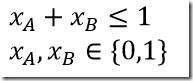(Note: we are smart and do not generate both xA+xB≤1 and xB+xA≤1) The test if two intervals A=[a1,a2], B=[b1,b2] overlap is: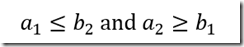or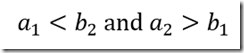depending on borderline cases (the last version is called the “strict” version in the following). Here is the demonstration: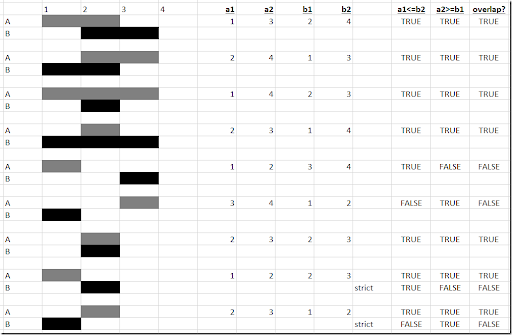Note that if the intervals are determined endogenously in the model, we often write “no-overlap” constraints for two intervals A=[a1,a2], B=[b1,b2] as:I.e. B starts after A ends or A starts after B ends. Indeed, we can see that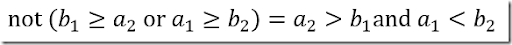which derives our second “strict” form of the overlap conditions.